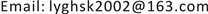JEEJournal of Electrical Engineering2333-5394Scientific Research Publishing10.12677/JEE.2018.63031JEE-26934JEE20180300000_58757413.pdf工程技术 基于合成矢量的MOA故障判断方法 Calculation Method of MOA Resistive Fundamental Current Growth Rate Based on Synthetic Vector 1*光宇1212许林12海荣32思坤12立位12国网江苏省电力公司连云港供电公司，江苏 连云港南京导纳能科技有限公司，江苏 南京null280820180603264269© Copyright 2014 by authors and Scientific Research Publishing Inc. 2014This work is licensed under the Creative Commons Attribution International License (CC BY). http://creativecommons.org/licenses/by/4.0/

MOA，合成矢量，相间耦合电容，阻性泄漏电流，相角变化, MOA Synthetic Vector Phase Coupling Capacitors Resistive Leakage Current Phase Angle Changes

1国网江苏省电力公司连云港供电公司，江苏 连云港

2南京导纳能科技有限公司，江苏 南京Copyright © 2018 by authors and Hans Publishers Inc.

This work is licensed under the Creative Commons Attribution International License (CC BY).

http://creativecommons.org/licenses/by/4.0/1. 引言

2. 数学模型

{ I ′ ˙ a = I ˙ a + I ˙ b a c I ′ ˙ b = I ˙ b + I ˙ a b + I ˙ c b = I ˙ b − I ˙ b a c I ˙ ′ c = I ˙ c + I ˙ b a c (1)

3. 阻性基波电流对总泄漏电流基波幅值和相位的影响

arctan ( I c ( 1 + k % ) I r ) 。由于阻性基波电流在总泄露电流中的占比一般为10%~20%，其增长变化对总泄露电

4. 耦合电容对总泄漏电流幅值和相角的影响

Resistive current increases by 100%, the magnitude of the leakage current and the rate of change of phase angl

0.3980.0350.4000.00085.0000%
0.3980.0380.4000.08084.5030.585
0.3980.0420.4010.16784.0071.169
0.3980.0450.4010.26283.5111.751
0.3980.0490.4010.36483.0172.333
0.3980.0520.4020.47482.5242.913
0.3980.0560.4020.59182.0313.492
0.3980.0590.4030.71581.5404.070
0.3980.0630.4030.84781.0514.646
0.3980.0660.4040.98680.5625.221
0.3980.0700.4051.13380.0755.794

5. 合成矢量

{ I ˙ a − c = I ′ ˙ a − I ˙ ′ c = I ˙ a + I ˙ b a c − ( I ˙ c + I ˙ b a c ) = I ˙ a − I ˙ c I ˙ a + b = I ˙ ′ a + I ˙ ′ b = I ˙ a + I ˙ b a c + I ˙ b − I ˙ b a c = I ˙ a + I ˙ b I ˙ c + b = I ˙ ′ c + I ˙ ′ b = I ˙ c + I ˙ b a c + I ˙ b − I ˙ b a c = I ˙ c + I ˙ b (2)

Coupling capacitance of the total leakage current amplitude and phase angle of the rate of chang
I ˙ b a c 有效/mAI ′ ˙ a 幅度变化/mAΔA/˚I ˙ ′ b 的幅度变化/mAΔB/˚I ˙ ′ c 的幅值变化/mAΔC/˚
0.010−0.004−1.312−0.010−0.128−0.0061.190
0.020−0.008−2.651−0.020−0.263−0.0112.415
0.030−0.012−4.015−0.030−0.405−0.0163.673
0.040−0.015−5.406−0.040−0.555−0.0224.967
0.050−0.018−6.821−0.050−0.713−0.0266.294
0.060−0.021−8.259−0.060−0.881−0.0317.657
0.070−0.024−9.719−0.070−1.058−0.0369.054
0.080−0.027−11.200−0.080−1.247−0.04010.485

The increase in resistive current leads to a table of composite vector angle change
Δ α /˚Δ β /˚Δ γ /˚
A相阻性电流增加100%−0.339−1.955−2.294
B相阻性电流增加100%3.008−1.0161.992
C相阻性电流增加100%−2.6693.0870.419

6. 结论